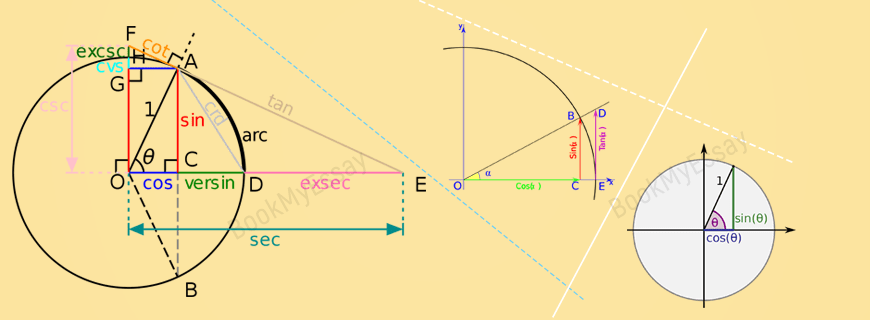# Trigonometry Assignment HelpGet a Free Quote
It's free and always will be.
Page - +
words
NWL

## Trigonometry Assignment Help

BookMyEssay is the most preferred online assignment help service that provides highly professional trigonometry assignment help along with all other allied service. Trigonometry is an important branch of mathematics that deals with the relationships between lengths and angles in a triangle. This branch of mathematics is used in different other applied fields of science, like in mechanics, astronomy, and different streams of engineering. Application of trigonometry is also quite usual in applied mathematics too, like in differential calculus and geometry. Students get their primary knowledge on trigonometry in high schools, and later while pursuing undergraduate courses they learn various applications of trigonometry more intensively.

### What is Trigonometry?

Simply speaking, trigonometry is a methodology for getting some unknown elements in a triangle, and other geometric shapes, provided sufficient related data are available on angular measurements and lengths to find out the missing data, e.g. if the two sides of a triangle is given and the angle they include is known, then the length of the third side can be measured with the help of law of cosines, and the angle the third side make with the other two sides can be measured with the help of law of sines.

Originally, trigonometry was used to define relations between any two elements of a triangle. In a triangle, there are six basic elements, i.e. 3 sides and 3 angles. The line segments that may serve as the three sides of a triangle, if they are capable to satisfy the triangle inequality, or rather three triangle inequalities. Similarly, not any three arbitrary angles may be the angles of a triangle. These necessities impose restrictions on the way in which the relations between the two elements in a triangle may be defined. In modern trigonometry, these relations are extended to any arbitrary angles also. This can be done, for example, by observing the projections of a rotating radius of a circle and a tangent at the end of the radius.

If the three sides of a triangle, a, b, c lie opposite to the angles α, β, γ respectively, then a + b > c is the inequality that the three sides of a triangle obey, and the summation of three angles, α + β + γ = 180° is the identity that holds in Euclidean geometry.

There are a hundreds of trigonometric identities available for different applications in different purposes. The most basic one is the following, where Pythagorean theorem a² + b² = c² is expressed in terms of sine and cosine:

sin² α + cos² α = 1.
Some other important identities are as follows –

1. sin 2α = 2 sin α cos α
2. cos 2α = cos² α – sin² α
3. tan 2α = 2 tan α / (1 – tan² α)
4. cot 2α = (cot² α – 1) / 2cot α.
5. sin (α + β) = sin α cos β + cos α sin β
6. cos (α + β) = cos α cos β – sin α sin β
7. sin (α – β) = sin α cos β – cos α sin β
8. cos (α – β) = cos α cos β + sin α sin β.

### Trigonometric Assignment Writing Help

This branch of mathematics is very vast and its application, as stated before, is very wide. Students are given assignments depending on their levels of studies like high school, undergraduate studies, postgraduate studies, etc.

Some common topics from which assignments are often given at undergraduate level are as follows –

• The Law of Cosines (Cosine Rule)
• Cosine of 36 degrees
• Tangent of 22.5 degrees
• Sine and Cosine of 15 Degrees Angle
• Sine, Cosine, and Ptolemy’s Theorem
• Morley’s Miracle
• Napoleon’s Theorem
• A Trigonometric Solution to a Difficult Sangaku Problem
• Trigonometric Form of Complex Numbers
• Derivatives of Sine and Cosine
• ΔABC is right iff sin²A + sin²B + sin²C = 2
• Hunting Right Angles
• Point on Bisector in Right Angle
• Trigonometric Identities with Arctangents
• The Concurrency of the Altitudes in a Triangle – Trigonometric Proof
• Butterfly Trigonometry
• Binet’s Formula with Cosines
• Another Face and Proof of a Trigonometric Identity
• cos/sin inequality

These and many other topics are quite commonly used in assignments and students sometimes fall in grave problem while handling their assignments on trigonometry.

The math assignment writing experts associated with BookMyEssay could be approached to get professional Trigonometric assignment writing help in this matter.

These experts accomplish any type of trigonometric assignments promptly and accurately within the due dates. Some other mention worthy aspects in this service is as follows –

• The service is very much affordable for the students.
• BookMyEssay provides 24/7 students help service.
• On demand emergency assignment help service.
• Amendment and rectification service without any extra charges.
• Scope to communicate with the writers to get latest updates.

Students are immensely benefited through this service since it saves much time and enables them to submit their assignments within due time.